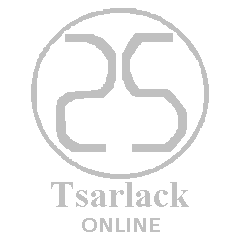City: Ashburn
Region: Virginia
Country: United States

Longitude: -77.4903
Latitude: 39.0469

Your Local DMA Code is 511

United States Currency Code: USD
Currency Symbol: \$
Ts€ Exchange Rate: 1.0773

Places near Ashburn:

Ashburn, Virginia

Dulles Town Center, Virginia

Sterling, Virginia

Herndon, Virginia

Poolesville, Maryland

GBCT Exchange Rates: Ts€ to \$

Ts€ 1 = \$1.08
Ts€ 5 = \$5.39

Ts€ 10 = \$10.77
Ts€ 15 = \$16.16
Ts€ 20 = \$21.55
Ts€ 25 = \$26.93
Ts€ 30 = \$32.32
Ts€ 35 = \$37.71
Ts€ 40 = \$43.09
Ts€ 45 = \$48.48
Ts€ 50 = \$53.87
Ts€ 55 = \$59.25
Ts€ 60 = \$64.64
Ts€ 65 = \$70.02
Ts€ 70 = \$75.41
Ts€ 75 = \$80.8
Ts€ 80 = \$86.18
Ts€ 85 = \$91.57
Ts€ 90 = \$96.96
Ts€ 95 = \$102.34

Ts€ 100 = \$107.73
Ts€ 200 = \$215.46
Ts€ 300 = \$323.19
Ts€ 400 = \$430.92
Ts€ 500 = \$538.65
Ts€ 600 = \$646.38
Ts€ 700 = \$754.11
Ts€ 800 = \$861.84
Ts€ 900 = \$969.57

Ts€ 1,000 = \$1077.3
Ts€ 5,000 = \$5386.5
Ts€ 10,000 = \$10773
Ts€ 25,000 = \$26932.5
Ts€ 50,000 = \$53865
Ts€ 75,000 = \$80797.5

Ts€ 100,000 = \$107730
Ts€ 250,000 = \$269325
Ts€ 500,000 = \$538650
Ts€ 750,000 = \$807975

Ts€ 1,000,000 = \$1077300
Ts€ 1,000,000,000 = \$1077300000